﻿ 《微波传输与接收实验》课程启发式教学应用

# 《微波传输与接收实验》课程启发式教学应用Heuristic Teaching in Microwave Transmission and Reception Experiments Course

Abstract: The heuristic teaching is introduced into the experimental course Microwave Transmission and Reception Experiments. It is mainly introduced in two teaching contents: one is to allow students to freely explore and research common microwave radiation sources in life, and the other is to explore the absorbing effects of common materials through teacher guidance. Practice has proved that the introduction of heuristic teaching makes students more involved and active in the classroom, and can better stimulate students' exploratory learning.

1. 引言

2. 《微波传输与接收实验》教学内容及教学设计简介

2.1. 教学内容

${S}_{0}=\frac{{P}_{\Sigma }}{4\text{π}{r}^{2}}\text{W}/{\text{m}}^{2}$ (1)

${S}_{0}=\frac{1}{2}\mathrm{Re}\left(E×{H}^{*}\right)=\frac{{|{E}_{0}|}^{2}}{240\text{π}}$ (2)

${E}_{0}=\frac{\sqrt{60{P}_{\Sigma }}}{r}$ (3)

${E}_{0}=\frac{\sqrt{60{P}_{i}{G}_{i}}}{r}$ (4)

${P}_{r}={S}_{0}\cdot {A}_{e}=\frac{{P}_{i}{G}_{i}}{4\text{π}{r}^{2}}\cdot \frac{{\lambda }^{2}{G}_{r}}{4\text{π}}$ (5)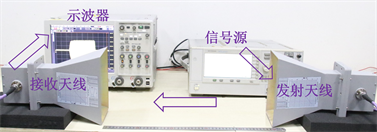Figure 1. Schematic diagram of experimental principle

2.2. 教学环节设计

3. 启发式教学

3.1. 微波辐射源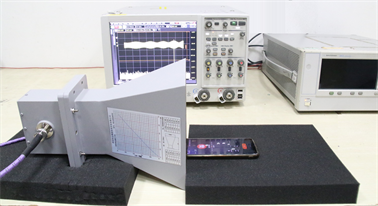Figure 2. Microwave radiation source (phone call) measurement(a) 某电信号码通话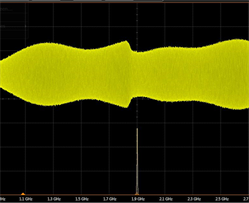(b) 某联通号码通话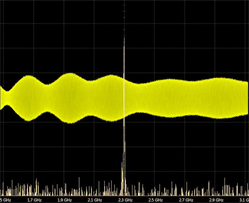(c) 某移动号码通话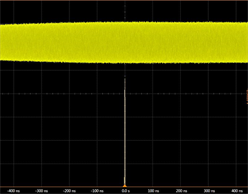(d) 蓝牙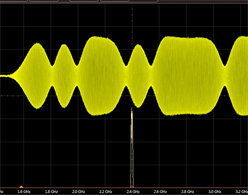(e) 上网

Figure 3. Typical waveforms of radiation during mobile phone calls, bluetooth, and internet

3.2. 吸波材料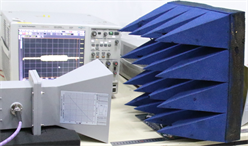(a) 吸波材料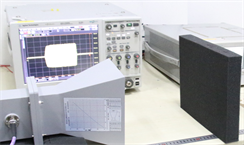(b) 黑海绵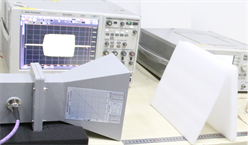(c) 白海绵

Figure 4. Schematic diagram of absorbing characteristics

4. 总结

 Benford, J., Swegle, J.A. and Schamiloglu, E. (2007) High Power Microwaves. Taylor & Francis Group, New York, 42-45, 206-207.
https://doi.org/10.1201/9781420012064

 顾茂章, 张克潜. 微波技术[M]. 北京: 清华大学出版社, 1989: 62-67.

 Kraus, J.D. and Marhefka, R.J. (2017) Antennas: For All Applications. Publishing House of Electronics Industry, Beijing, 26-27.

Top